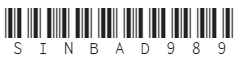# Elements of Set Theory: Arithmetic

in mathematics •  2 years ago

# Arithmetic

We can apply recursion theorem, from the previous section, to define addition and multiplication on.

Suppose we want a functionsuch thatis the result of adding 5 to n. Thenmust satisfy the conditionsThe recursion theorem assures us that a unique such function exists.

`Definition` A binary operation on a set A is a function frominto A.

`Definition` Addition (+) is the binary operation onsuch that for any m and n in.Thus when written as a relationIn conformity to everyday notation, we write m+n instead of.

`Theorem 4I` For natural numbers m and n,This theorem is an immediate consequence of the construction of. The Theorem 4I serve to characterize the binary operation + in a recursive fashion.

We now proceed to construct the multiplication operation in much the same way.

`Definition` Multiplicationis the binary operation onsuch that for any m and n in,This is just an extension of Theorem 4I.

`Theorem 4J` For natural numbers m and n,For convenience, we can now discard thefunctions, and useand use Theorem 4J instead.

We could, in the same manner, define the exponentiation operation onThe equations that characterize exponentiation are,`Example` Show 2 + 2 = 4

We can show the addition as follow:We have given some set-theoretic definitions of the operations of arithmetic, the next step is to verify that some of the common laws of arithmetic are provable within set theory.

`Theorem 4K` The following identities hold for all natural numbers.(3) Distributive law(4) Associative law for multiplication(5) Commutative law for multiplicationDisclaimer: this is a summary of section 4.4 from the book "Elements of Set Theory" by Herbert B. Enderton, the content apart from rephrasing is identical, most of the equations are from the book and the same examples are treated. All of the equation images were screenshots from generated latex form using typora